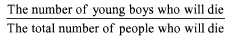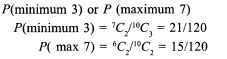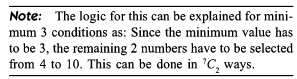Courses

# Test: Probability- 5

## 15 Questions MCQ Test General Aptitude for GATE 2020 | Test: Probability- 5

Description
This mock test of Test: Probability- 5 for UPSC helps you for every UPSC entrance exam. This contains 15 Multiple Choice Questions for UPSC Test: Probability- 5 (mcq) to study with solutions a complete question bank. The solved questions answers in this Test: Probability- 5 quiz give you a good mix of easy questions and tough questions. UPSC students definitely take this Test: Probability- 5 exercise for a better result in the exam. You can find other Test: Probability- 5 extra questions, long questions & short questions for UPSC on EduRev as well by searching above.
QUESTION: 1

### The ratio of number of officers and ladies in the Scorpion Squadron and in the Gunners Squadron are 3 : 1 and 2 : 5 respectively. An individual is selected to be the chairperson of their association. The chance that this individual is selected from the Scorpions is 2/3. Find the probability that the chairperson will be an officer

Solution:

(2/3) x (3/4) + (1/3) x (2/7) = (1/2) + (2/21) = (25/42)

QUESTION: 2

### A speaks the truth 3 out o f 4 times, and B 5 out o f 6 times. What is the probability that they will contradict each other in stating the same fact?

Solution:

They will contradict each other if: A is true and B is false or A is false and B is true.

(3/4) x (1/6) + (1/4) x (5/6) = 1/3.

QUESTION: 3

### If 4 whole numbers are taken at random and multiplied together, what is the chance that the last digit in the product is 1, 3, 7 or 9?

Solution:

The whole numbers selected can only be 1, 3, 7 or 9 and cannot contain 2, 4, 6, 8, 0 or 5.

QUESTION: 4

A life insurance company insured 25,000 young boys, 14,000 young girls and 16,000 young adults. The probability of death within 10 years of a young boy, young girl and a young adult are 0.02, 0.03 and 0.15 respectively. One of the insured persons dice. What is the probability that the dead person is a young boy?

Solution:

The required probability will be given by the expression:QUESTION: 5

In a bag there are 12 black and 6 white balls. Two balls are chosen at random and the first one is found to be black. The probability that the second one is also black is:

Solution:

11/17 (if the first one is black, there will be 11 black balls left out of 17)

QUESTION: 6

Three of the six vertices of a regular hexagon are chosen at random. The probability that the triangle with these vertices is equilateral is

Solution:

There will be 6C3 triangles formed overall. Out of these visualise the number of equilateral triangles.

QUESTION: 7

A pair of fair dice are rolled together till a sum of either 5 or 7 is obtained. The probability that 5 comes before 7 is

Solution:

We do not have to consider any sum other than 5 or 7 occurring.
A sum of 5 can be obtained by any of [4 + 1, 3 + 2, 2 + 3, 1 + 4]
Similarly a sum of 7 can be obtained by any of [6 + 1, 5 + 2, 4 + 3, 3 + 4, 2 + 5, 1 + 6]
For 6: n(E) = 4, n(S) = 6 + 4 P = 0.4
For 7: n(E) = 6 n(S) = 6 + 4 P = 0.6

QUESTION: 8

Out of a pack of 52 cards one is lost; from the remainder of the pack, two cards are drawn and are found to be spades. Find the chance that the missing card is a spade.

Solution:

This problem has to be treated as if we are selecting the third card out of the 50 remaining cards. 11 of these are spades.
Hence, 11/50.

QUESTION: 9

Counters marked 1, 2, 3 are placed in a bag and one of them is withdrawn and replaced. The operation being repeated three times, what is the chance of obtaining a total of 6 in these three operations?

Solution:

A total o f 6 can be obtained by either (1 + 2 + 3) or by (2 + 2 + 2).

QUESTION: 10

The probability of a bomb hitting a bridge is 1/2 and two direct hits are needed to destroy it. The least number of bombs required so that the probability of the bridge being destroyed is greater than 0.9 is:

Solution:

Try to find the number of ways in which 0 or 1 bomb hits the bridge if n bombs are thrown.
The required value of the number of bombs will be such that the probability of 0 or 1 bomb hitting the bridge should be less than 0.1.

QUESTION: 11

In the above question, find the probability that the remaining two balls are red.

Solution:

The required probability would be given by the event definition: First is red and second is red = 5/22 x 4/21 = 10/231

QUESTION: 12

A bag contains 15 tickets numbered 1 to 15. A ticket is drawn and replaced. Then one more ticket is drawn and replaced. The probability that first number drawn is even and second is odd is

Solution:

In the first draw, we have 7 even tickets out of 15 and in the second we have 8 odd tickets out of 15.
Thus, (7/15) x (8/15) = 56/225.

QUESTION: 13

AMS employs 8 professors on their staff. Their respective probability of remaining in employment for 10 years are 0.2, 0.3, 0.4, 0.5, 0.6, 0.7, 0.8, 0.9. The probability that after 10 years at least 6 of them still work in AMS is

Solution:

Event definition: Any six of them work AND four leave OR any seven work AND three leave OR any eight work AND two leave OR any nine work AND one leaves OR All ten work.

QUESTION: 14

Three numbers are chosen at random without replacement from (1, 2, 3 ..., 10). The probability that the minimum number is 3 or the maximum number is 7 is

Solution:QUESTION: 15

If from each of three boxes containing 3 white and 1 black, 2 white and 2 black, 1 white and 3 black balls, one ball is drawn at random, then the probability that 2 white and 1 black ball will be drawn is

Solution:

Two white and one black can be obtained only through the following three sequences: Ball drawn from A and B are white and the ball drawn from C is Black. or Ball drawn from A and C are white and the ball drawn from B is Black. or Ball drawn from B and C are white and the ball drawn from A is Black.